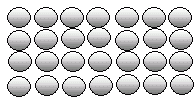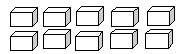Name: ___________________Date:___________________

 Email us to get an instant 20% discount on highly effective K-12 Math & English kwizNET Programs!

### Grade 2 - Mathematics7.9 Multiplication Review Test

 Q 1: Write the multiplication form for 4 + 4 + 4 + 44 + 4 = 84 X 3 = 124 X 4 = 16 Q 2: Use the figure to find 4 times 7.282625 Q 3: When two numbers are multiplied the answer is calledproductsumdifference Q 4: 0 x 5 =01015 Q 5: 5 x 8 =45323540 Q 6: What is the multiplication form for: 3+3+3+33 x 3 = 93 x 4 = 124 x 4 = 16 Q 7: Use the figure to find 5 times 2.121011 Q 8: Write the multiplication form for 13 + 13 + 1313 X 1 = 1313 X 3 = 3913 + 3 = 16 Question 9: This question is available to subscribers only! Question 10: This question is available to subscribers only!

#### Subscription to kwizNET Learning System costs less than \$1 per month & offers the following benefits:

• Unrestricted access to grade appropriate lessons, quizzes, & printable worksheets
• Instant scoring of online quizzes
• Progress tracking and award certificates to keep your student motivated
• Unlimited practice with auto-generated 'WIZ MATH' quizzes